### D.47 Born dif­fer­en­tial cross sec­tion

This note de­rives the Born dif­fer­en­tial cross sec­tion of ad­den­dum {A.30}.

The gen­eral idea is to ap­prox­i­mate (A.228) for large dis­tances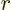. Then the as­ymp­totic con­stant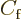in (A.216) can be iden­ti­fied, which gives the dif­fer­en­tial cross sec­tion ac­cord­ing to (A.218). Note that the Born ap­prox­i­ma­tion took the as­ymp­totic con­stant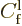equal to one for sim­plic­ity.

The main dif­fi­culty in ap­prox­i­mat­ing (A.228) for large dis­tancesis the ar­gu­ment of the ex­po­nen­tial in the frac­tion. It is not ac­cu­rate enough to just say that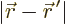is ap­prox­i­mately equal to. You need the more ac­cu­rate ap­prox­i­ma­tion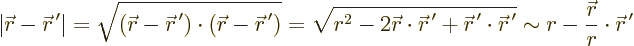The fi­nal ap­prox­i­ma­tion is from tak­ing a fac­tor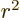out of the square root and then ap­prox­i­mat­ing the rest by a Tay­lor se­ries. Note that the frac­tion in the fi­nal term is the unit vec­tor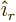in the-​di­rec­tion.

It fol­lows that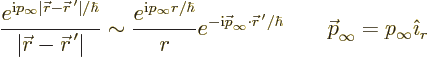Also, in the sec­ond ex­po­nen­tial, since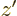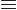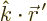,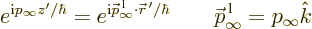Writ­ing out the com­plete ex­pres­sion (A.228) and com­par­ing with (A.216) gives the con­stantand hence the dif­fer­en­tial cross sec­tion.